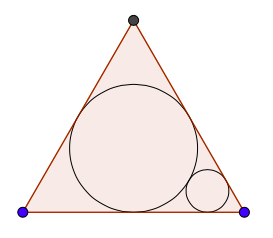# A geometric problem, part II

Geometry Level 2You have a circle (circle 1) inscribed in an equilateral triangle. Then, you construct another circle (circle 2) that is tangent to two sides of the triangle and to circle 1. Then, you construct another circle (circle 3) that is tangent to two sides of the triangle and to circle 2. Then you construct another circle (circle 4) that is tangent to two sides of the triangle and to circle 3. Then you keep doing this infinitely. Now, not only do you do this for one angle of the triangle, but you repeat the same process for the other two angles of the triangle.

By doing the above, you get an infinite number of circles. If the radius of the circle 2 is 1, and if the area covered by all of those circles can be represented in the form $\dfrac{a \pi}{b}$, where $a$ and $b$ are coprime positive integers, find $a+b$.

×

Problem Loading...

Note Loading...

Set Loading...